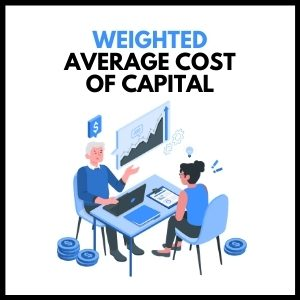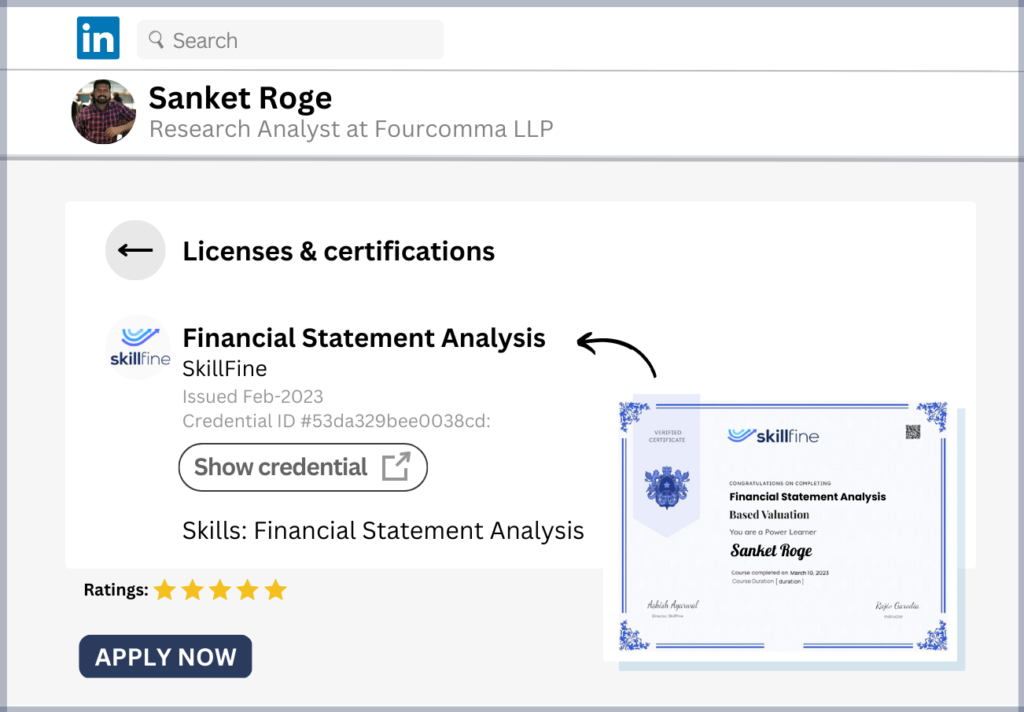# Weighted Average Cost of Capital (WACC): Definition and Calculation## Weighted Average Cost of Capital of a company

When we want to do valuation of any company using the Discounted Cash Flows valuation, we need to calculate a cost of capital.

The cost of capital is simply the expected return that investors (both debt and equity) expect from investing their money into the company.

The minimum expected return that investors expect from investing in a company is called the Weighted Average Cost of Capital (WACC).

Let us show you a formula on how to calculate the WACC of any company.Let us explain this further.

Typically, the capital of any company comprises Debt as well as Equity.

The proportion of Debt and Equity in the total capital will definitely vary amongst companies.

When we calculate the WACC, we need to take into account the return expectations of both Debt investors as well as Equity investors.

The return expectation of debt providers is calculated as Cost of Debt and the return expectation of equity providers is Cost of Equity.

We, of course, need to weight the return expectations of Debt and Equity with their respective proportion in the total capital.

The question then is how do we calculate the Cost of Debt and the Cost of Equity?

The cost of debt can be calculated in 2 different ways.

First, if the Debt of the company is currently listed and trades in the market as a bond, we can simply take the current long term (10+ years) Yield To Maturity (YTM) from the market.

The Yield to Maturity (YTM) is simply the current interest rate on a publicly traded debt (borrowings) of a company.

### From where can you find the data on the YTM of company debt?

Well, you can visit popular finance websites like Bloomberg where you can find the current Yield To Maturity for any publicly traded bonds of companies.

The second approach is applicable for companies who do not have publicly traded bonds.

In this approach, we simply divide the interest expense cost of the company by the total debt of the company.

This will provide us with the actual cost of debt of the company.

We use this to find the cost of debt of the company.

The one thing we should remember here is that for the calculation of WACC, we typically take the post-tax cost of debt.

That is, we take the cost of debt and multiply it with (1-tax rate).

So, if the cost of debt is 10% and tax rate of the country is 40%, then the post-tax cost of debt

is = 10%*(1-40%)

= 6%.

There are tax benefits to a company by taking on more debt hence, the actual cost to the company is lowered by the tax shields the company would get on it.

Using either of the two approaches, we can calculate the cost of debt for a company.

So far, clear?

Let us now come to Cost of Equity calculation.

The cost of equity can be calculated using the CAPM (Capital Asset Pricing Model) framework.

Let us explain what this framework is.

Let’s say you as an investor did not want to take any risk by investing your money in an asset.

That is, you want to get risk free return.

Now, which is that asset class that will provide you risk free return?

Clearly, you can invest in the government bonds i.e. sovereign bonds to get risk free returns.

Again, you can simply visit finance sites like Bloomberg , you can find out the current interest rates you earn by investing in the government bonds.

Typically, it is denoted by Rf and varies by the respective country where you would like to invest in.

For example, the 10-year US Government bonds are current trading at 2.83% and the 10-year Indian government bonds are trading at 7.91%.

Now, when you invest in Equity, clearly you are assuming a higher risk than the risk-free Government bonds.

Let us say, you invested in the broader market portfolio where the portfolio covers all the major stocks in that country.

Typically, there are Equity Indices that capture the broader market portfolio of any country.

In the US, it is typically the S&P 500 index and in India, it is the Nifty 50 index.

Every country has a representative index that provides a representation of the broader market portfolio.

The returns generated by the market index is denoted by Rm.

Now, you can easily find out the returns that is generated by an Index over a long-term horizon.

Once again if you go to Bloomberg you can find out the last 10 years return provided by the market in any of the respective country indices.

The S&P 500 has generated ~9% in the last 10 years (2008-2018) and the Nifty 50 has generated ~14% over the same time horizon.

So, it follows that if you invested in the risk-free US government bond you would earn ~3% and if you invested in the risky broader market portfolio in US, you would earn ~9%

So, the excess return over the risk-free rate, you earn while investing in the riskier broader US market portfolio is ~6% (9%-3%).

And the excess return you earn while investing in the riskier broader India market portfolio is ~6% (14%-8%)

[Disclaimer: This is purely historical empirical data. It is not an indication of the future returns. So take it more for explanatory purposes.]

This excess return is called Market Risk Premium (Rm-Rf).

Simply put, it is the excess return over the risk-free government bond that you can earn by investing in the broader market portfolio.

### Now, when you invest in a company, you need to assess the risk of investing in this company vs the broader market portfolio.

That is, we need to evaluate if the risk of investing in the company is more than the market risk or less than it?

This is typically evaluated using a metric called Equity Beta.

Equity Beta simply represents the relative risk of investing in a company vs the market portfolio.

The market portfolio is assumed to have a Equity Beta of 1.

So if the risk of investing in a company is more than the market risk, it will have an equity beta of more than 1.

For example, real estate companies will have a equity beta more than 1 since the business tends to do better than the overall economy in a high growth macro-economic environment and the opposite is also true.

And if the risk is less than the market portfolio risk, then the company will have an equity beta less than 1. Typically, FMCG companies tend to have a beta less than 1. They remain resilient to the overall business cycle in the economy.

### But how do you calculate Beta for a company?

The beta of a company can be simply calculated by regressing the historical returns of the company vs the overall market returns.

You take the monthly returns of the company stock price over the last 10-20 years and take the monthly returns of the market portfolio index over the same horizon.

Now, simply do a linear regression of these returns to find out the slope co-efficient of the regression equation.

The slope co-efficient is the Equity Beta of the company.

It denotes how the returns in company stock move in effect to movement in the overall market portfolio.

### What has this to do with cost of equity calculation?

To answer this, let us begin to bring together all the pieces here.

If you invest in a risk-free asset, you can expect to earn Rf (risk free rate) as returns.

If you invest in a broader market portfolio you can expect Rm.

In other words, you can also say that by investing in the market portfolio, you will earn

= Rf + (Rm-Rf)

That is, you earn the risk-free rate + the market risk premium for a market portfolio with a beta of 1.

Now, if you invest in a company with let say beta of B, then the corresponding return you can expect is

Rf + (Rm-Rf) * B

Where B denotes the beta of the company relative to the broader market portfolio.

So if B is more than 1, you will earn higher than Rm and if B is less than 1 you earn less than Rm.

This therefore becomes the cost of equity for a company.

Numerical example:

Let us say, we have US retailer which has 20% debt and 80% equity in the total capital.

The tax rate is 40%.

The 10 year government bond is trading at 3%.

The current yields to maturity (YTM) of 10 year US retailer company bond is 5%.

The equity beta of the company is 0.9x.

And the 10-year market return in S&P 500 has been 9%.

### So what is the WACC of this US retailer company?

Using the formula above, let us first calculate the post-tax cost of debt.

It is

= 5% * (1 – 40%)

= 3%

Cost of Equity is

= 3% + 0.9 * (9% – 3%)

= 8.4%

The Weighted Average Cost of Capital is

= (20% * 3%) + (80% * 8.4%)

= 7.3%

Makes sense?